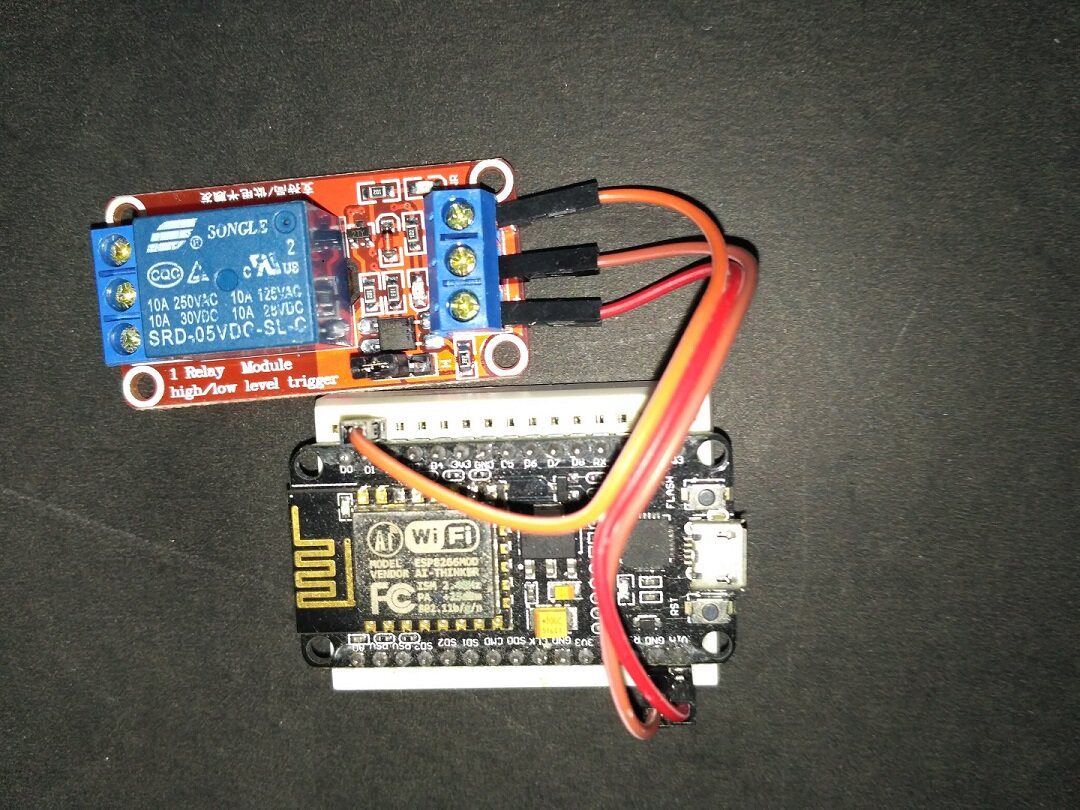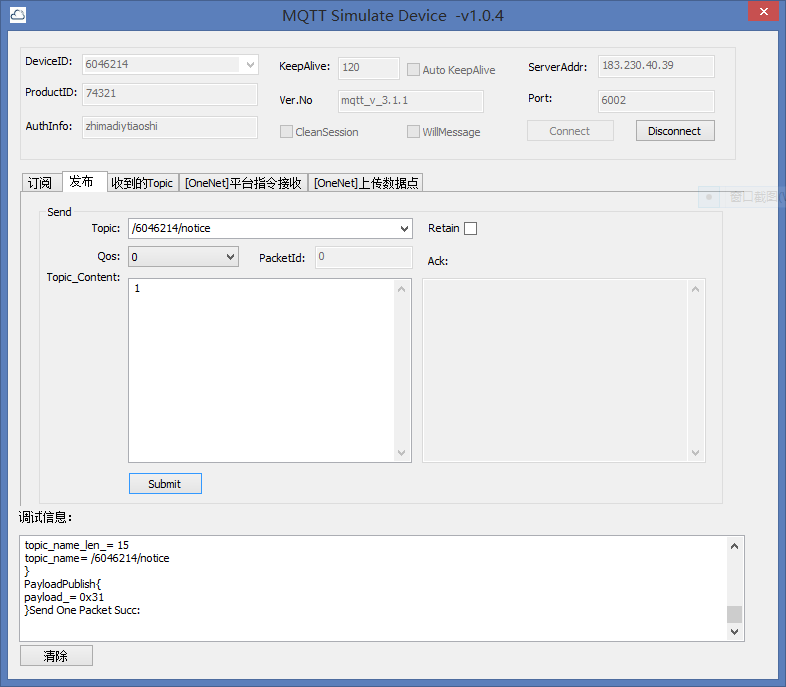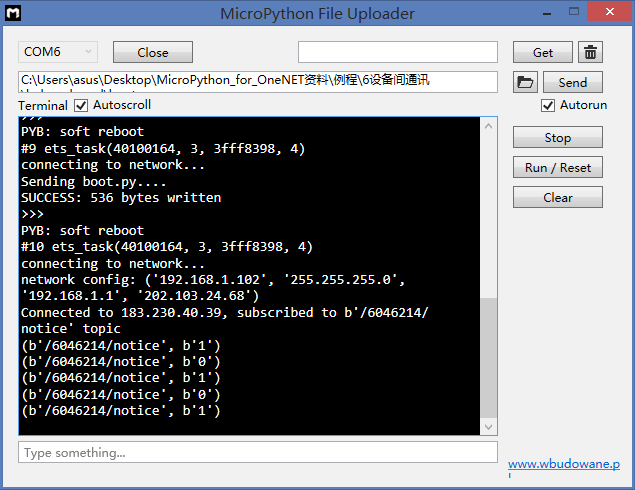# MicroPython:通过OneNET平台控制继电器```def do_connect(): import network sta_if = network.WLAN(network.STA_IF) ap_if = network.WLAN(network.AP_IF) if ap_if.active(): ap_if.active(False) if not sta_if.isconnected(): print('connecting to network...') sta_if.active(True) sta_if.connect('MERCURY_D5C8', 'zhimadiy') #wifi的SSID和密码 while not sta_if.isconnected(): pass print('network config:', sta_if.ifconfig()) do_connect() gc.collect()```

``` from umqtt.simple import MQTTClient from machine import Pin import ubinascii import machine import micropython led = Pin(5, Pin.OUT, value=1) #MQTT服务端信息，使用时修改成自己的 SERVER = "183.230.40.39" #ONENET服务器地址 SERVER_PORT=6002 #ONENET服务器端口 CLIENT_ID = "3784858" #创建设备时得到的设备ID，为数字字串 TOPIC = b"/6046214/notice" #通过订阅 /device_id/数据流名 的方式，及时获取到某设备最新的数据点信息。 username='74321' #注册产品时，平台分配的产品ID，为数字字串 password='zhimadiymicropythonesp8266' #鉴权信息 state = 0 def sub_cb(topic, msg): global state print((topic, msg)) if msg == b"0": led.value(0) state = 1 elif msg == b"1": led.value(1) state = 0 elif msg == b"toggle": # LED is inversed, so setting it to current state # value will make it toggle led.value(state) state = 1 - state def main(server=SERVER): #括号里主要是客户端ID，服务器地址，服务器端口，用户产品ID，鉴权信息 c = MQTTClient(CLIENT_ID, server,6002,username,password) # Subscribed messages will be delivered to this callback c.set_callback(sub_cb) c.connect() c.subscribe(TOPIC) print("Connected to %s, subscribed to %s topic" % (server, TOPIC))```

``` try: while 1: #micropython.mem_info() c.wait_msg() finally: c.disconnect() ```

`main()`

TOPIC = b”/6046214/notice”